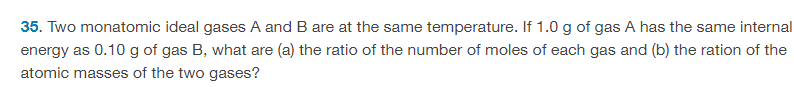# Ratio of atomic masses of two ideal gases

• ChiralSuperfields

#### ChiralSuperfields

Homework Statement
Relevant Equations
For part(b)The solution is, ##1:10##, however, is the wording correct? I don't see how to find the ratio of atomic mass, however, I can solve for the ratio of the molar mass.

##n_A = n_B## from part(a) by setting the internal energy equation for each ideal gas equal
##\frac{M_A}{m_A} = \frac{M_B}{m_B}##
##1000M_A = 10000M_B##
##M_A = 10M_B##

Is the reason they said that is because ##u ∝ M##. Is there a equation that proves that?

Many thanks!

What is the definition of Avagadro's number?

•ChiralSuperfields
What is the definition of Avagadro's number?

It is the number of molecules per 1 mol. So has units ##\frac{1}{mol}##

Many thanks!

I think there is also a spelling error in part(b) it should be ratio not ration

What is the definition of Avagadro's number?

Many thanks!

Last edited:
It might be better to write it as ##\frac {number~ of~ molecules}{mole}## in this circumstance

•ChiralSuperfields
It might be better to write it as ##\frac {number~ of~ molecules}{mole}## in this circumstance
Thank you for your reply @hutchphd! I agree that is a much better way to write it. However, is the wording to this problem in post #1 wrong? Or dose Avagadro's number relate to this in some way.

Many thanks!

I found from dimensional analysis that the I can get correct units for a mass of one atom (atomic mass):

## \frac{M_A}{N_A} = 10\frac{M_B}{N_A} ##
## u_A = 10u_B##